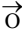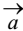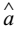# Types of Vectors

### Zero or Null Vector

A vector whose initial and terminal points are coincident is called a zero or null or a void vector. The zero vector is denoted by.

Vectors other than the null vector are called proper vectors.

### Unit Vector

A vector whose modulus is unity, is called a unit vector.

The unit vector in the direction ofis denoted by(read as "a cap").

### Like and Unlike Vectors

Vectors are said to be like when they have the same sense of direction and unlike when they have opposite directions.

### Co-initial Vectors

Vectors having the same initial point are called co-initial vectors.

### Co-terminus Vectors

Vectors having the same terminal point are called co-terminus vectors.

### Collinear or Parallel Vectors

Vectors are said to be collinear or parallel if they have the same line of action or have the lines of action parallel to one another.

### Coplanar Vectors

Vectors are said to be coplanar if they are parallel to the same plane or they lie in the same plane.

### Negative Vector

The vector which has the same magnitude as that ofbut opposite direction is called the negative vector and is denoted by −.

### Reciprocal of a Vector

The vector which has the same direction but has magnitude reciprocal to that of a given vector.